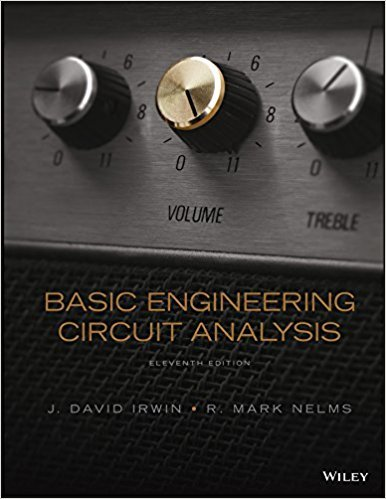×
Get Full Access to Basic Engineering Circuit Analysis - 11 Edition - Chapter 6 - Problem 6.75
Get Full Access to Basic Engineering Circuit Analysis - 11 Edition - Chapter 6 - Problem 6.75

×

# Find the value of L in the network in Fig. P6.75 so thatISBN: 9781118539293 159

## Solution for problem 6.75 Chapter 6

Basic Engineering Circuit Analysis | 11th Edition

• Textbook Solutions
• 2901 Step-by-step solutions solved by professors and subject experts
• Get 24/7 help from StudySoup virtual teaching assistantsBasic Engineering Circuit Analysis | 11th Edition

4 5 1 399 Reviews
15
3
Problem 6.75

Find the value of L in the network in Fig. P6.75 so that the value of L T will be 2 mH. 4 mH 1 mH 2 mH 6 mH LT L L Figure P6.75

Step-by-Step Solution:
Step 1 of 3

Step 2 of 3

Step 3 of 3

##### ISBN: 9781118539293

Unlock Textbook Solution